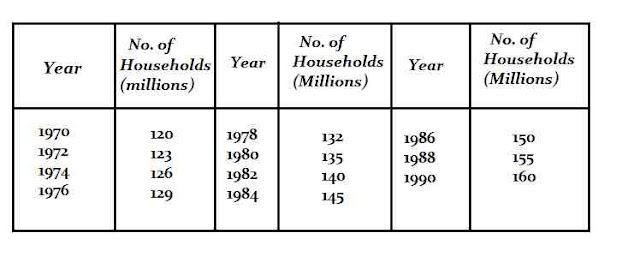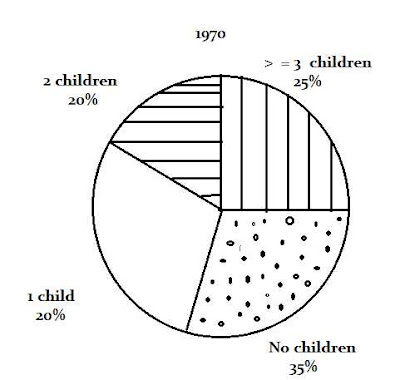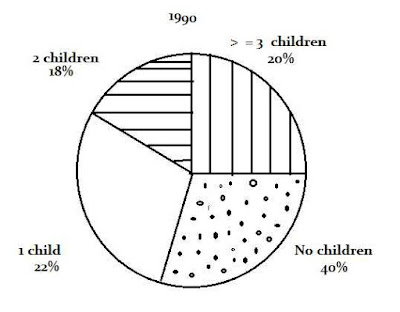# Important Data Interpretation Set for SBI Clerk Mains

Today I am sharing an important Data Interpretation Set for SBI Clerk Mains.

### Directions:

The following table gives the number of households in a country during the period 1970-90.The following pie charts give the distribution of households based on the number of children for the year 1970 and 1990.#### Ques 1.

Which of the following statements about the households is true ?
(a) There were more households with children in 1970 than in 1990.
(b) There were more households with 3 or more children in 1970 than in 1990.
(c) The number of households with two children decreased slightly from 1970 to 1990
(d) None of the above
Ans 1. No. of households  with children in 1970
= ( 100-35/100 x 120) million = 78 million
No. of households with children in 1990
= ( 100-40/100 x 160) million = 96 million
Hence, statement (a) is not true.
No. of households with 3 or more children  in 1970
=(25/100 x 120) million = 30 million
No. of households with 3 or more children  in 1990
= (20/100 x 160) million = 32 million
Hence, statement (b) is not true.
No. of households with 2 children in 1970
= ( 20/100 x 120)million = 24 million
No. of households with 2 children in 1990
= ( 18 /100 x 160) Million = 28.8 million
Hence, statement (C) is not true
Thus, statement (d) is true.

#### Ques 2.

The simple annual growth rate of number of households from 1970 to 1990 was
(a) 1.52%
(b) 1.65%
(c) 2.10%
(d) 3.00%
Ans 2.  The required growth rate
= ( 160-120/120 x20 x 100)% = 1.65%( approx).

#### Ques 3.

The average number of children per households in 1990 was
(a) 1.18
(b) 1.35
(c) 2.0
(d) Cannot be determined
Ans 3. The average number of children per house hold  cannot be  determined since one of the categories of households is ' households with >  = 3 children'. In other words, we do not know the exact average figure for this category .Hence, the overall average cannot be worked out.

#### Ques 4.

Assuming that the average number of children per households in 1970 was, how many households were without any children in 1970 ?
(a) 24 million
(b) 42 million
(c) 14 million
(d) Cannot be determined
Ans 4. No. of households without any children
in 1970 =m ( 35/100 x 120) million
= 42.0 million.